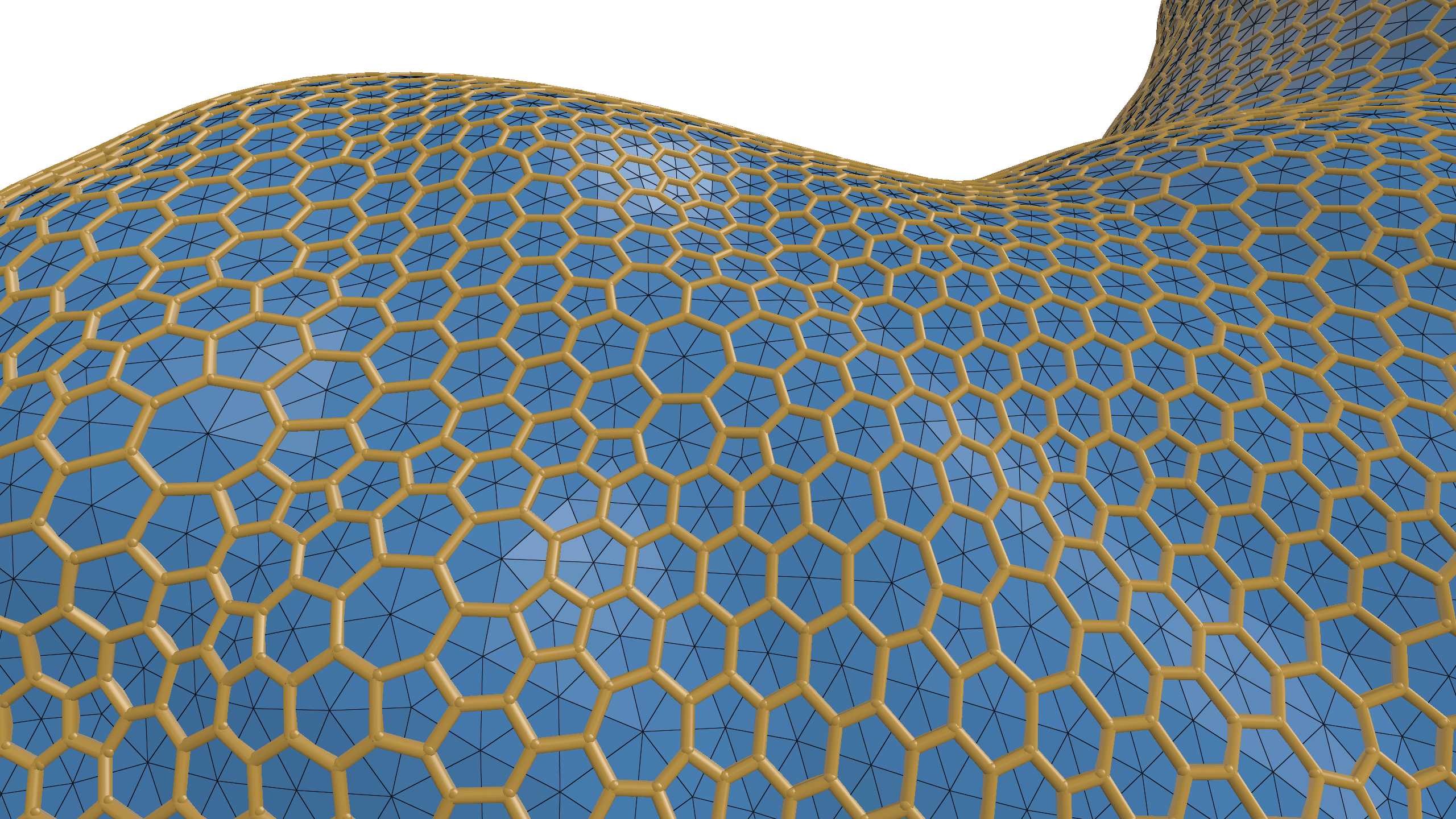# Misc Quantities

### Surface Graph Quantity

The surface graph quantity is a collection of nodes and straight-line edges between them. Each node is given as a position in 3D space—this quantity does not really have any relationship to the underlying surface, execpt that it is managed as a surface quantity.

Nodes will be drawn as spheres, and the connecting edges drawn as cylinders. This quantity can be useful for visualizing paths on the surface, or wireframes.

Example: drawing mesh’s dual with geometry-central.#include "polyscope/surface_mesh.h"

// geometry-central things
geom->requireFaceIndices();

std::vector<Vector3> positions;
std::vector<std::array<size_t, 2>> edgeInds;

// Build the node positions
for (Face f : mesh->faces()) {

// Compute center for face
Vector3 c = Vector3::zero();
for (Vertex v : f.adjacentVertices()) {
c += geom->inputVertexPositions[v];
}
c /= f.degree();

positions.push_back(c);
}

// Build the edge indices
for (Edge e : mesh->edges()) {

// Connect the nodes from the two faces adjacent to each edge
size_t fa = geom->faceIndices[e.halfedge().face()];
size_t fb = geom->faceIndices[e.halfedge().twin().face()];

edgeInds.push_back({fa, fb});
}

polyscope::getSurfaceMesh("my mesh")->

SurfaceMesh::addSurfaceGraphQuantity(std::string name, const P& nodes, const E& edges)

Add a new surface graph quantity to the structure.

• nodes is the list of 3D positions for the graph nodes. The type should be adaptable to a list of float-valued 3-vectors.
• edges is the list of edges for the graph, where each entry is two 0-based indices in to the nodes array. The type should be adaptable to a list of size_t-valued 2-vectors (aka pairs of indices).

The resulting class has color and radius fields which can be set to adjust the appearance of the resulting graph.

### Options

Parameter Meaning Getter Setter Persistent?
enabled is the quantity enabled? bool isEnabled() setEnabled(bool newVal) yes
radius the radius the graph is drawn with double getRadius() setRadius(double val, bool isRelative=true) yes
color the color to draw the graph with glm::vec3 getVectorColor() setVectorColor(glm::vec3 val) yes

(all setters return this to support chaining. setEnabled() returns generic quantity, so chain it last)